Register for Maths, Science, English, Reasoning Olympiad Exams Register here | Book Free Demo for Live Olympiad Classes here | Check Olympiad Exam Dates here | Buy Practice Papers for IMO, IOM, HEO, IOEL etc here | Login here to participate in all India free mock test on every Saturday

# Number Name and Sense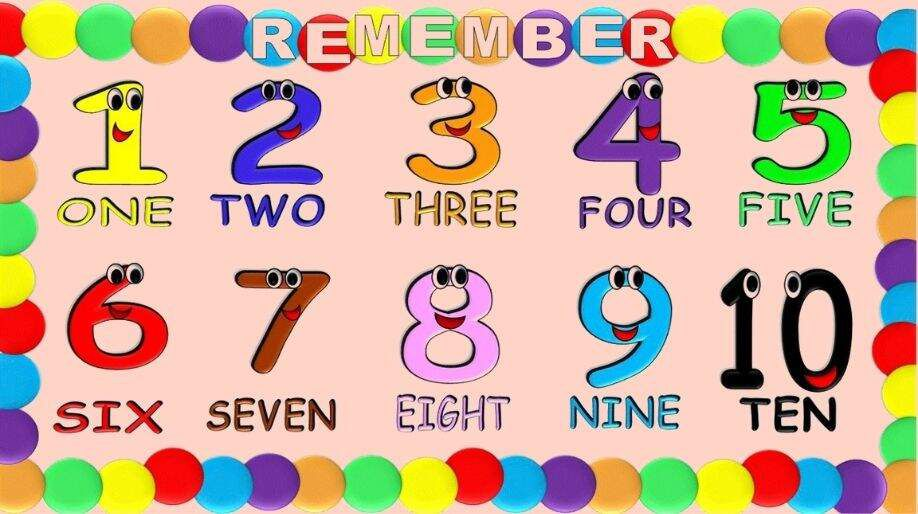Numbers are the basic unit of mathematics. A number system is a writing system for expressing numbers. Do you want to know how things are measured, counted or compared? Let's find out!

The places ones, tens, and hundred together are called one's period

 One's Period Hundreds Tens Ones 0 0 1 0 3 2 4 5 6

• The numbers at One's place are named as:

1 = One
2 = Two
3 = Three
4 = Four
5 = Five
6 = Six
7 = Seven
8 = Eight
9 = Nine

The numbers at Tens place are named as:
• 10 = Ten
20 = Twenty
30 = Thirty
40 = Forty
50 = Fifty
60 = Sixty
70 = Seventy
80 = Eighty
90 = Ninety
• The numbers at Hundred’s place are named as:

100 = One hundred
200 = Two hundred
300 = Three hundred
400 = Four hundred
500 = Five hundred
600 = Six hundred
700 = Seven hundred
800 = Eight hundred
900 = Nine hundred

# Write the number in words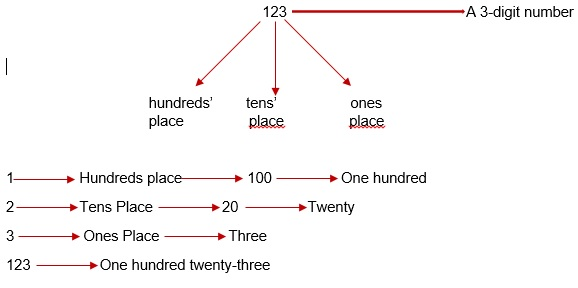# Guess the numbers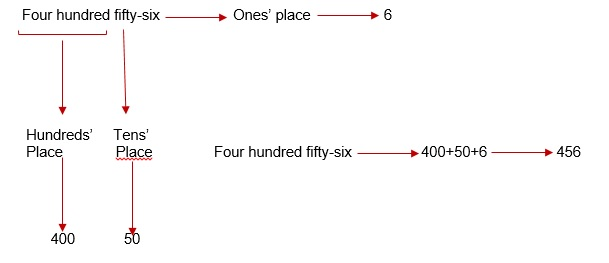# Practice these questions

Q1) In the box given below match the words to the numbers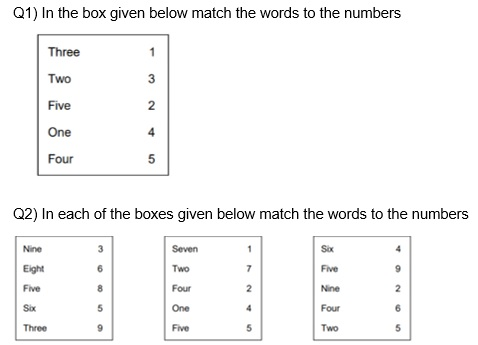Q3) Count the hundreds, tens, and ones and write the numbers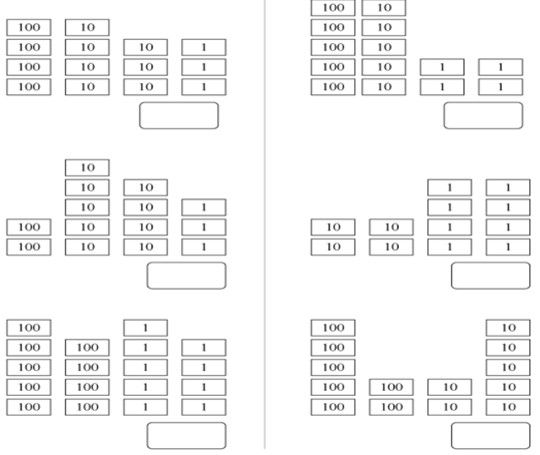Q4) Write the following number in expanded form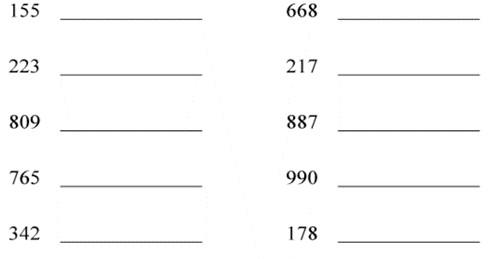Q5) Write the following number in standard form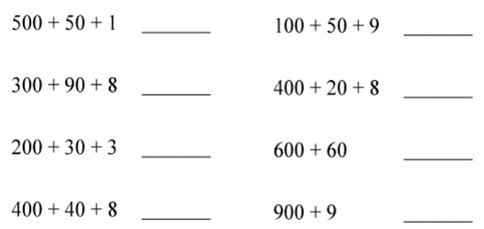Q6) Write the value of the underlined digits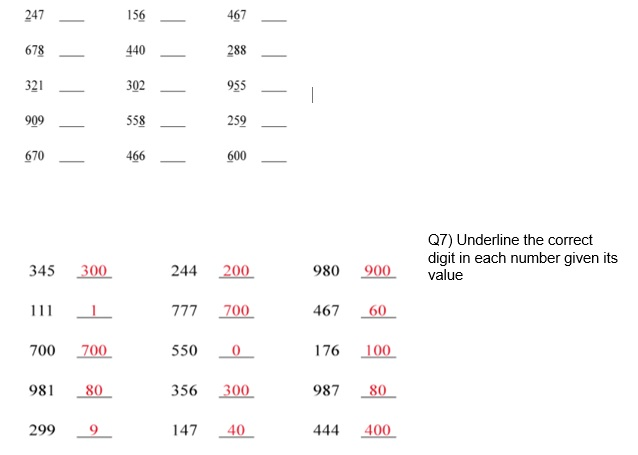Q8) Identify the numbers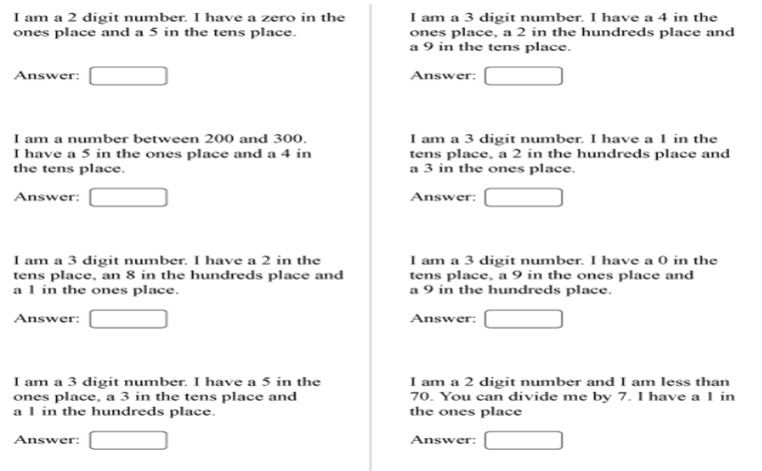## Quiz for Number Name and Sense

 Q.1 How many blue stars can you see?a) 4 b) 3 c) 2 d) 1
 Q.2 Which is the greatest number among "25, 10, 56, 08, 97"? a) 10 b) 97 c) 8 d) 56
 Q.3 Number name for "679". a) Six seven nine b) Sixty sevennine c) Sixseventy nine d) Six hundred seventy nine
 Q.4 Which is the smallest number among " 124, 463, 402, 281, 356, 122, 211"? a) 211 b) 122 c) 124 d) 281
 Q.5 Which is the smallest 3-digit nunber? a) 100 b) 10 c) 102 d) 000
 Q.6 Which is the largest 3-digit number? a) 100 b) 896 c) 999 d) 998
 Q.7 Number of green gifts in this picture are _____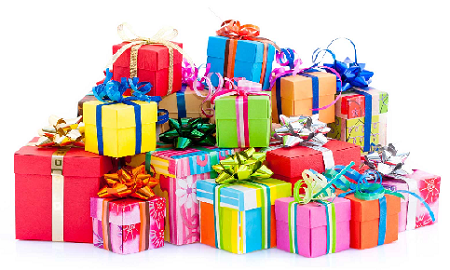a) 4 b) 2 c) 3 d) 8
 Q.8 How many pastries can you see?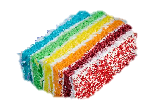a) 3 b) 2 c) 1 d) 4
 Q.9 How many pineapples are there?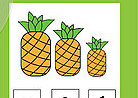a) 2 b) 5 c) 4 d) 3
 Q.10 The place value of "1" in "415" is _____ a) tens b) hundreds c) ones d) thousands